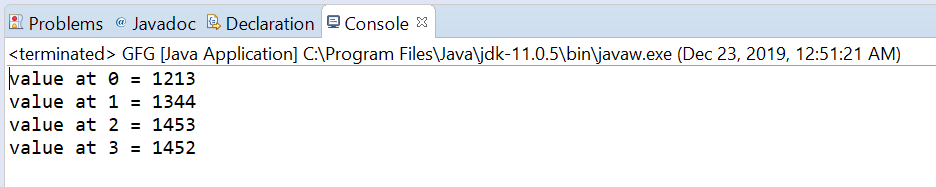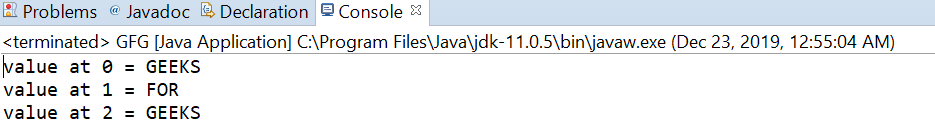Related Articles

# AtomicReferenceArray getAcquire() method in Java with Examples

• Last Updated : 03 Jan, 2020

The getAcquire() method of a AtomicReferenceArray class is used to return the value of the element at index i for this AtomicReferenceArray object with memory ordering effects compatible with memory_order_acquire ordering.

Syntax:

```public final E getAcquire(int i)
```

Parameters: This method accepts the index i to get the value.

Return value: This method returns current value at index i.

Below programs illustrate the getAcquire() method:
Program 1:

 `// Java program to demonstrate``// AtomicReferenceArray.getAcquire() method`` ` `import` `java.util.concurrent.atomic.AtomicReferenceArray;`` ` `public` `class` `GFG {``    ``public` `static` `void` `main(String[] args)``    ``{`` ` `        ``// create an atomic reference array``        ``// object which stores Integer.``        ``AtomicReferenceArray array``            ``= ``new` `AtomicReferenceArray(``5``);`` ` `        ``// set some value in array``        ``array.set(``0``, ``1213``);``        ``array.set(``1``, ``1344``);``        ``array.set(``2``, ``1453``);``        ``array.set(``3``, ``1452``);`` ` `        ``// get and print the value``        ``// using getAcquire method``        ``for` `(``int` `i = ``0``; i < ``4``; i++) {`` ` `            ``int` `value = array.getAcquire(i);``            ``System.out.println(``"value at "``                               ``+ i + ``" = "` `+ value);``        ``}``    ``}``}`
Output:Program 2:

 `// Java program to demonstrate``// AtomicReferenceArray.getAcquire() method`` ` `import` `java.util.concurrent.atomic.AtomicReferenceArray;`` ` `public` `class` `GFG {``    ``public` `static` `void` `main(String[] args)``    ``{`` ` `        ``// create an atomic reference array object``        ``// which stores String.``        ``AtomicReferenceArray array``            ``= ``new` `AtomicReferenceArray(``5``);`` ` `        ``// set some value in array``        ``array.set(``0``, ``"GEEKS"``);``        ``array.set(``1``, ``"FOR"``);``        ``array.set(``2``, ``"GEEKS"``);`` ` `        ``// get and print the value using get method``        ``for` `(``int` `i = ``0``; i < ``2``; i++) {`` ` `            ``String value = array.getAcquire(i);``            ``System.out.println(``"value at "``                               ``+ i + ``" = "` `+ value);``        ``}``    ``}``}`
Output:Attention reader! Don’t stop learning now. Get hold of all the important Java Foundation and Collections concepts with the Fundamentals of Java and Java Collections Course at a student-friendly price and become industry ready. To complete your preparation from learning a language to DS Algo and many more,  please refer Complete Interview Preparation Course.

My Personal Notes arrow_drop_up